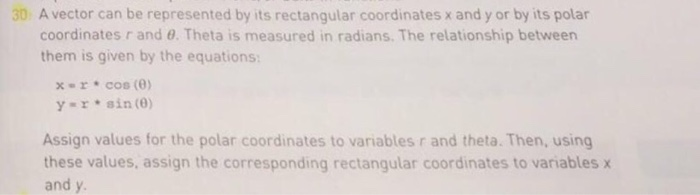# Question & Answer: A vector can be represented by its rectangular coordinates x and y or by its polar coordinates r and theta. Theta is me…..I need it done by Matlab

A vector can be represented by its rectangular coordinates x and y or by its polar coordinates r and theta. Theta is measured in radians. The relationship between them is given by the equations: x = x * cos (theta) y = r * sin (theta) Assign values for the polar coordinates to variables r and theta. Then, using these values, assign the corresponding rectangular coordinates to variables x and y.

the following program describes that how to convert polar coordinates to rectangular coordinates, In this i have taken 9 polar ponts that is 9 theta values and 9 r values.

Don't use plagiarized sources. Get Your Custom Essay on
Question & Answer: A vector can be represented by its rectangular coordinates x and y or by its polar coordinates r and theta. Theta is me…..
GET AN ESSAY WRITTEN FOR YOU FROM AS LOW AS \$13/PAGE

program:–

theta=0:pi/4:2*pi; %theta values starts from 0 to 2pi witha an intervel of pi/4 total 9 values assigning to theta
r=1:1:9;    % r values taken as 1 to 9 (1,2,3,4,5,6,7,8,9)
fprintf(‘taken polar coordinates are:n’);
for i=1:1:9
fprintf(‘(%f,%f)n’,r(i),theta(i));   %printing r,theta value pairs as polar coordinates
end
%conver to rectangular coordinates
x=r.*cos(theta);    %assigning x values total 9 x values
y=r.*sin(theta);    %assigning y values total 9 y values

fprintf(‘nncorsponding rectangular coordinates are:n’);
for i=1:1:9
fprintf(‘(%f,%f)n’,x(i),y(i));   %printing x,y value pairs as rectangular coordinates
end

output:–

rect_polar_coordinate
taken polar coordinates are:
(1.000000,0.000000)
(2.000000,0.785398)
(3.000000,1.570796)
(4.000000,2.356194)
(5.000000,3.141593)
(6.000000,3.926991)
(7.000000,4.712389)
(8.000000,5.497787)
(9.000000,6.283185)

corsponding rectangular coordinates are:
(1.000000,0.000000)
(1.414214,1.414214)
(0.000000,3.000000)
(-2.828427,2.828427)
(-5.000000,0.000000)
(-4.242641,-4.242641)
(-0.000000,-7.000000)
(5.656854,-5.656854)
(9.000000,-0.000000)